Get instant live expert help with Excel or Google Sheets“My Excelchat expert helped me in less than 20 minutes, saving me what would have been 5 hours of work!”

#### Post your problem and you’ll get expert help in seconds.

Your message must be at least 40 characters
Our professional experts are available now. Your privacy is guaranteed.

# Excel NORMSINV Function – Excelchat

NORMSINV is a built-in function in Excel under Statistical Functions that returns the inverse of the standard normal cumulative distribution, which has a zero mean and a standard deviation value “1”.

NORMSINV is the inverse of the function NORMDIST and is compatible with Excel version 2007 and earlier.  Newer versions of Excel from 2010 to present features an updated function, NORM.S.INV.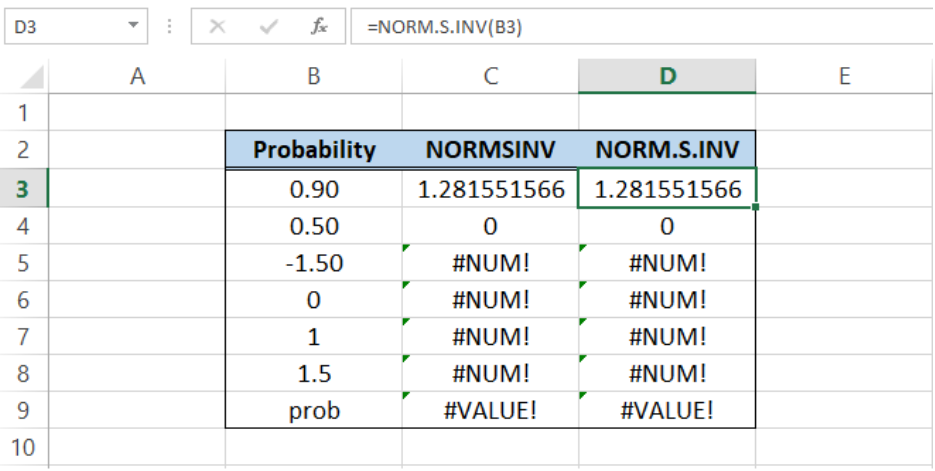Figure 1.  Final result:  NORMSINV and NORM.S.INV function

## Syntax of NORMSINV and NORM.S.INV

`=NORMSINV(probability)  `

`=NORM.S.INV(probability)  `

• where probability is greater than zero “0”  and less than 1
• When probability<=0 or probability>=1, the function returns the error value #NUM!
• When probability has a non-numeric value, the function returns the error #VALUE!

## Examples

Calculate the inverse of the standard normal cumulative distribution with a probability of 0.90.

We simply enter the following formulas:

In cell D3: `=NORMSINV(0.90)`

In cell F3: `=NORM.S.INV(B3)`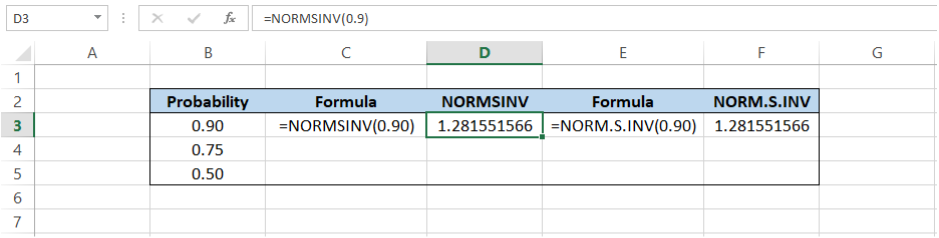Figure 2.  Entering the formula

Note that both functions have the same results.  When we apply the functions for the succeeding probability values 0.75 and 0.50, the results are shown below.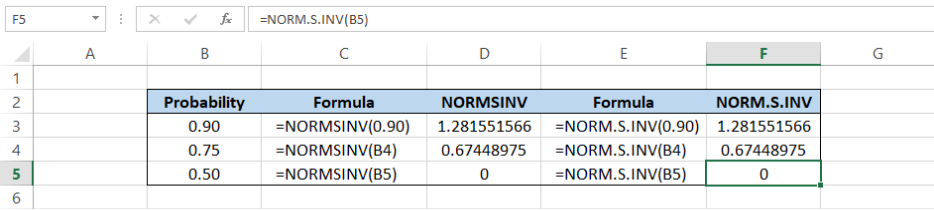Figure 3.  Output: NORMSINV and NORM.S.INV functions

NORM.S.INV is an updated version of the function NORMSINV and in some key calculations, NORM.S.INV will be able to provide results with greater accuracy.

## Common Errors

There are two common errors encountered with these functions:

• A probability value less than or equal to zero, or a value greater than or equal to 1 will result to a #NUM! Error
• A non-numeric value for probability will result to a #VALUE! error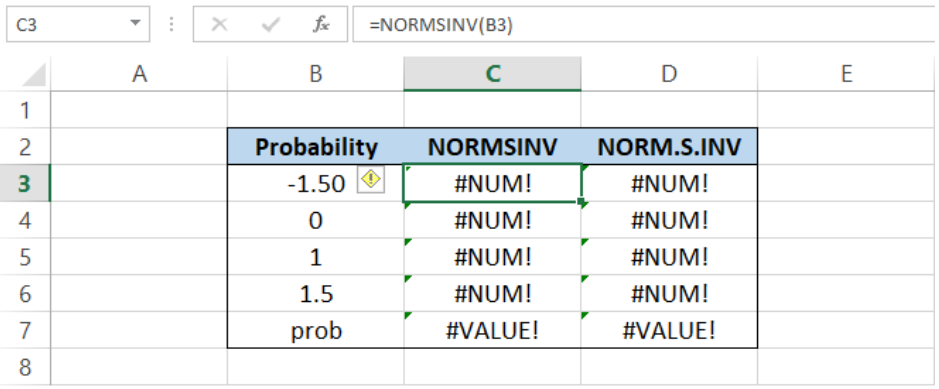Figure 4.  Common errors

The function can also be accessed through the ribbon under the Formulas tab > More Functions button > Statistical > NORM.S.INV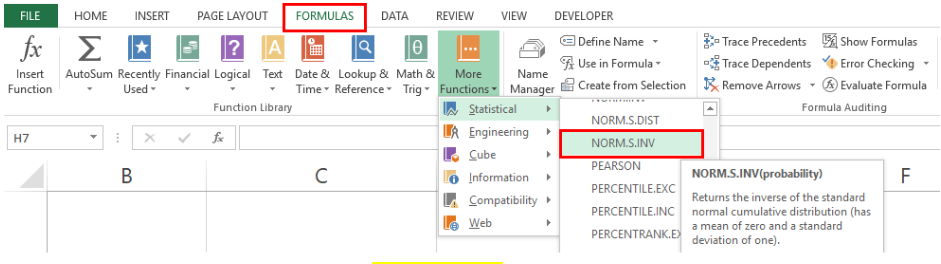Figure 5.  NORM.S.INV in menu options

## Instant Connection to an Excel Expert

Most of the time, the problem you will need to solve will be more complex than a simple application of a formula or function. If you want to save hours of research and frustration, try our live Excelchat service! Our Excel Experts are available 24/7 to answer any Excel question you may have. We guarantee a connection within 30 seconds and a customized solution within 20 minutes.